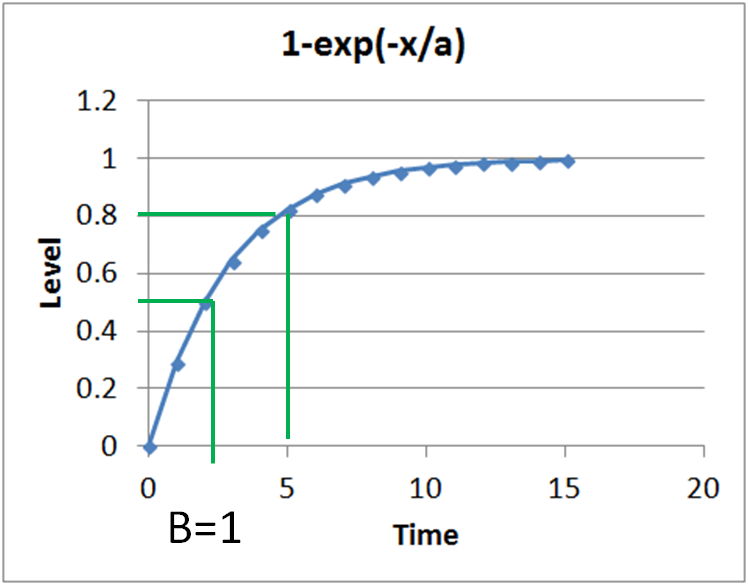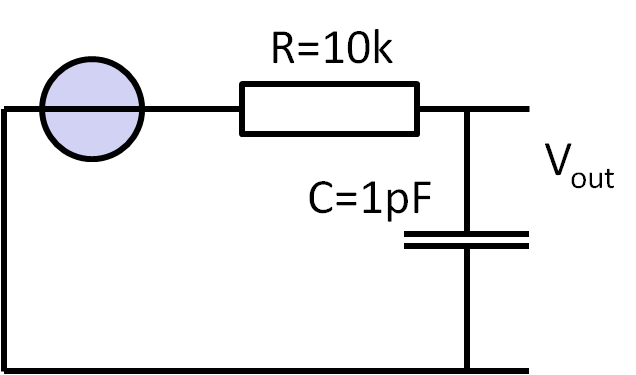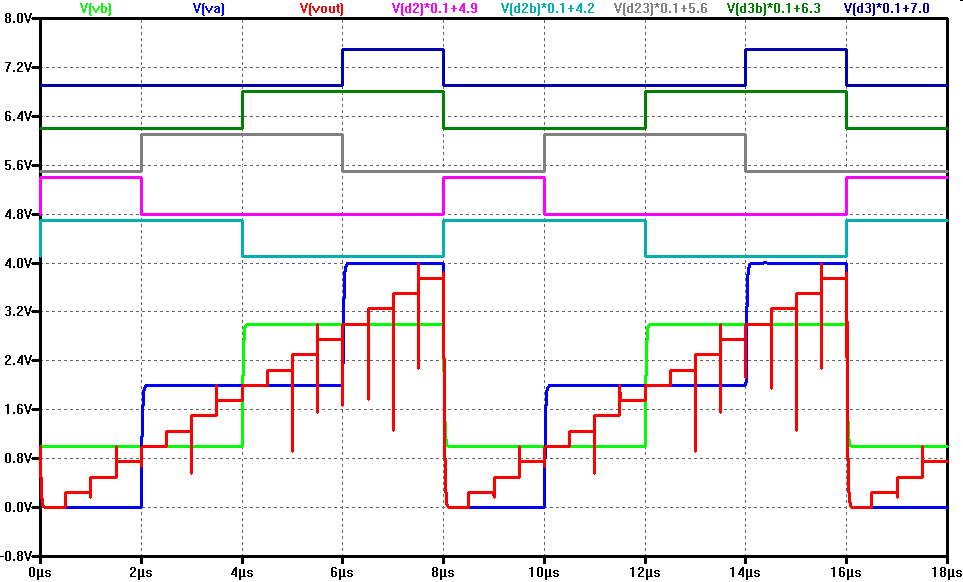05 DAC Architectures

04 Spectral Test

Video Lecture: DAC architectures

 Video is not visible, most likely your browser does not support HTML5 video Lõnge: 01:06:27 0:00:00 Laboratory specify your .raw file directory 0:03:29 Internet copyright form 0:04:18 Laboratory 3 start 0:07:28 DAC Architecture 0:08:02 DAC Performance 0:10:51 DAC Speed voltage difference requirements 0:16:36 RC Low pass thermal noise and LSB 0:20:15 Minimum C requirement C > 12 k T 22B/VFS2 0:21:26 Maximum speed R requirement 0:23:13 RC Low pass thermal noise capacitance requirement 0:25:26 Capacitance and white noise 0:26:45 3 bit R string or ladder DAC 0:29:50 Code 011 output voltage 0:32:38 Trnasfer curve calculation 0:33:32 Speed, power, numbe rof bits, complexity 0:36:13 Power 0:39:00 Interpolating R-string DAC 0:45:39 R2R DAC 0:48:21 Equivalent voltage source 0:50:36 2nd stage D1 0:55:10 DAC in Webreport for laboratory 2 0:57:27

Review and Overview

• Performance
• DAC architectures
• R string DAC
• Interpolating DAC
• Parallel charge scaling DAC
• Equivalent sources
• Split array charge scaling DAC
• Realization of capacitances
• R2R DAC
• Example: R-string DAC
• Statistical errors: INL and DNL

Performance

Go from one voltage level to another between certain sampling times.
At the moment we assume an ideal sample and hold circuit. Real sample and hold circuits are presented later.

A simple RC charging circuit is our model.

Performance parameters are:
• Speed: Sampling rate, bandwidth, latency
• Power
• Number of bits
• Complexity

DAC speed: voltage difference requirement

 A capacitor has to be charged to the 1/2 LSB range of the input voltage: $| V_e - V_a | < 0.5 LSB$ The voltage on the capacitor is: $V_a = V_e \cdot \left( 1 - e^{-\frac{t}{\tau}} \right)$ with $\tau = RC$ Maximum difference could be full scale $V_e = 2^{B} \cdot LSB$ with B number of bits: $2^{B} \cdot LSB \cdot e^{-\frac{t}{\tau}} < 0.5 LSB$ $-\frac{t}{\tau} < \left( -B-1 \right) ln \left( 2 \right)$ $t > R C \left( B+1 \right) ln \left( 2 \right)$Measurement: Time when half level is reached: t1
Settling time: (B+1)*t1

A capacitor has to be charged to the 1/2 LSB range of the input voltage:
$| V_e - V_a | < 0.5 LSB$
The voltage on the capacitor is:
$V_a = V_e \cdot \left( 1 - exp^{-\frac{t}{\tau}} \right)$ with $\tau = RC$
This gives:
$V_e - V_e \cdot \left( 1 - exp^{-\frac{t}{\tau}} \right) < 0.5 LSB$       $V_e \cdot e^{-\frac{t}{\tau}} < 0.5 LSB$
Maximum difference could be full scale $V_e = 2^{B} \cdot LSB$ with B number of bits:
 $2^{B} \cdot LSB \cdot exp^{-\frac{t}{\tau}} < 0.5 LSB$ $exp^{-\frac{t}{\tau}} < 2^{-B-1}$ $-\frac{t}{\tau} < ln \left( 2^{-B-1} \right)$ $-\frac{t}{\tau} < \left( -B-1 \right) ln \left( 2 \right)$ $t > R C \left( B+1 \right) ln \left( 2 \right)$

Measurement:
Time when half level is reached: t1
Settling time: (B+1)*t1

RC low pass thermal noise

 A resistor has a noise voltage: $\frac{V_{rms}^2}{\Delta f} = 4 k_B \cdot T \cdot R$ A low pass RC network limits the noise to: $\overline{v_n^2} = \frac{k_B T}{C}$ This noise has to be lower than the quantization noise: $\overline{v_q^2} = \frac{\Delta^2}{12} = \frac{V_{FS}^2}{2^{2B} \cdot 12}$ $\frac{k_B T}{C} \lt \frac{V_{FS}^2}{2^{2B} \cdot 12}$ $\frac{C}{k_B T} \gt \frac{2^{2B} \cdot 12}{V_{FS}^2}$Wikipedia: Thermal noise, Johnson, Nyquist noise
Elektronik 3: 24 Rauschen

RC low pass thermal noise capacitance requirement

$C \gt 12 \cdot k_B \cdot T \frac{2^{2 B}}{V_{FS}^{2}}$
kB: Boltzmann constant
T: absolute temperature in Kelvin
B: number of Bits
VFS: Full scale voltage
 B Cmin(VFS=3.3V) Cmin(VFS=1V) 8 0.3 fF 0.003 pF 12 80 fF 0.8 pF 16 20.6 pF 206 pF 20 5.28 nF 52.8 nF 24 1.32 uF 13.2 uF
Table: Required C as function of ADC resolution and full scale voltage
$\sqrt{\frac{k_B T}{C}} = \sqrt{\frac{V_{FS}^2}{2^{2B} \cdot 12}}$
 C $\sqrt{\frac{k_B T}{C}}$ 1fF 2mV 10fF 640 µV 100fF 200µV 1pF 64µV 10pF 20µV 100pF 6.4µV 1nF 2µV
Adding 4 bits required an increase in capacitance by a factor of 256.
Decreasing the voltage by a factor of 3.3 increases the capacitance with a factor of 10.

3 Bit R string or ladder DAC

 A R ladder divides VREF voltage into all possible voltage levels. Inherent monotonic Example: Input Code [d2 d1 d0] = 011 LSB = Vref/8 Vout = LSB * (0*4 + 1*2 + 1) = 3/8 Vref

3 Bit R string or ladder DAC

Speed

Time constant:
Ideal voltage source at a series RC low pass.
R = (3 R || 5 R) = 15/8 R
Maximum resistance for half VDD, code 100...

Power

Static
$P_{RS} = \frac{V_{ref}^2}{R \cdot 2^{B}}$

Number of bits

Resistance range:
1 Ω .. 1 MΩ
20 bits.
Resistance for MOSFET switches and contacts.

Complexity

2B resistors and 2 * 2B switches are a high element count
τ = 0.25 · 2B R C
There are 2B of unit resistors.
The highest resistance is at midpoint. Half resistance will be connected to VDD and half to ground.
For equivalent resistance these resistances are in parallel.

Practical example

M. Pelgrom, ōA 10-b 50-MHz CMOS D/A Converter with 75-W Buffer,ö JSSC, Dec. 1990, pp. 1347

1 V pp output into 75 Ω, 25 pF load
1.6 µm CMOS, 5 V; 65mW (50 MHz, 1Vpp); 1024 resistors 6..10 Ω
16 large resistors 250 Ω parallel to 32 75 Ω polysilison resistors with switches each;
Power: driver transistor 7.3 mA, driver circuit 4 mA; ladder current 1mA, digital 0.7 mA: total 13mA.
Rise fall time 6ns; 19 Mhz signal bandwidth; 50 MHz sample frequency; 2.5 mm2 die size
DNL; INL < 0.35; 5MHz Signal -53 dB to total harmonic distortion

Resolution: 8, 14, 20 bit
Frequency: 10 kHz, 1 MHz, 100 MHz
VDD = VREF = 1V (Integrated Circuits)

4 Bit interpolating R string, ladder DACExtra logic is needed to generate the control signals from the binary input code.
2 switches are always active on the left side for the 2 high order bits providing an upper and lower voltage for interpolation.
The simulation shows the control signals for the upper bits, the intermediate voltages VA and VB and the resulting output voltage Vout.

 MSBDA3 DA2 DA1 LSBDA0 D3 D3b D23 D2b D2 D1 D1b D01 D0 D0B VA/V VB/V 0 0 0 0 0 0 0 1 1 1 0 0 0 1 0 1

3 Bit binary charge scaling DAC

 Each capacitor is binary weighted. $V_{max} = V_{ref}$ Using equivalent current sources: $\underline{I}_{Di} = \underline{V}_{ref} \cdot j ω C_{i}$ $V(D2D1D0)= V_{ref} \frac{D2 \cdot C_{2} + D1 \cdot C_{1} + D0 \cdot C_{0} }{C_{Total}}$ $C_{Total} = \sum_{i=0}^N C_{i}$
This configuration can not generate any DC content.
Therefore reset switches are needed after each cycle.
Alternatively a code of all 0 can be applied between data output.

4 Bit binary split array charge DAC

 The range of C can be limited using a coupling capacitor C4.

R2R DAC

 Only R and 2R values are needed.
Calculation of output voltage with equivalent sources:
R is 1 kΩ.
All data inputs can be looked at as voltage sources VD0...VD3.
The voltages internally for equivalent sources are V0L..V2L, VoutL.
$V_{0L} = V_{D0} \frac{2 R}{4 R} = V_{D0} \frac{1}{2}$
$R_{i0} = 2 R || 2R = \frac{2 R 2 R}{2 R + 2 R} = R$
$V_{1L} = (V_{0L} - V_{D1}) \frac{2 R}{4 R} + V_{D1} = V_{0L} \frac{1}{2} + V_{D1} \frac{1}{2} = V_{D0} \frac{1}{4} + V_{D1} \frac{1}{2}$
$R_{i1} = 2 R || 2R = \frac{2 R 2 R}{2 R + 2 R} = R$

$V_{nL} = (V_{(n-1)L} - V_{Dn}) \frac{2 R}{4 R} + V_{Dn} = V_{(n-1)L} \frac{1}{2} + V_{Dn} \frac{1}{2} = \sum_{i=0}^{n} \frac{V_{Di}}{2^{n-i+1}}$
Capacitors can be used instead of the resistance R giving a C2C DAC.

Practical considerations

 Variations of components: R, C, V offset of operational amplifier What is allowed to have less than 1/2 LSB INL and DNL?

06 DAC Error

References

C. Lin and K. Buit, " A 10-b, 500- MSample/s CMOS DAC in 0.6mm2", JSSC, vol. 33, pp.1948-1958,1998

Untrimmed segmented
T. Miki et al, ōAn 80-MHz 8-bit CMOS D/A Converter,ö JSSC December 1986, pp. 983
A. Van den Bosch et al, ōA 1-GSample/s Nyquist Current-Steering CMOS D/A Converter,ö JSSC March 2001, pp. 315 Savoj, J, A 12-GS/s Phase-Calibrated CMOS Digital-to-Analog Converter for Backplane Communications , JSSC, 2008, pp. 1207 - 1216

Current copiers:
D. W. J. Groeneveld et al, ōA Self-Calibration Technique for Monolithic High-Resolution D/A Converters,ö JSSC December 1989, pp. 1517

Dynamic element matching:
R. J. van de Plassche, ōDynamic Element Matching for High-Accuracy Monolithic D/A Converters,ö JSSC December 1976, pp. 795